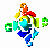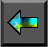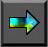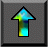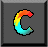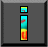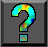Next: 6.1 FM Introduction Up: Part II: Tools Previous: Chapter Notes

# 6 Fortran M

In this chapter, we describe Fortran M (FM), a small set of extensions to Fortran for parallel programming. In FM, tasks and channels are represented explicitly by means of language constructs. Hence, algorithms designed using the techniques discussed in Part I can be translated into programs in a straightforward manner.

Because Fortran M is a simple language, we are able in this chapter to provide both a complete language description and a tutorial introduction to important programming techniques. (Some familiarity with Fortran is assumed.) In the process, we show how the language is used to implement various algorithms developed in Part I.

After studying this chapter, you should be able to write simple FM programs. You should know how to create tasks and channels, how to implement structured, unstructured, and asynchronous communication patterns, and how to control the mapping of tasks to processors. You should also know both how to guarantee deterministic execution and when it is useful to introduce nondeterministic constructs. Finally, you should understand how FM supports the development of modular programs, and know how to specify both sequential and parallel composition.Next: 6.1 FM Introduction Up: Part II: Tools Previous: Chapter Notes